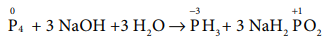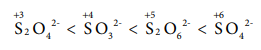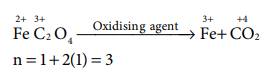# UNIT 1: Basic Concepts of Chemistry and Chemical Calculations - Online Test

Q1. Choose the disproportionation reaction among the following redox reactions.
Explaination / Solution:Q2. The equivalent mass of potassium permanganate in alkaline medium is MnO4- + 2H2O+3e-  MnO2 + 4OH-
Explaination / Solution:

The reduction reaction of the oxidising agent(MnO4) involves gain of 3 electrons.

Hence the equivalent mass = (Molar mass of KMnO4)/3 = 158.1/3 = 52.7

Q3. Which one of the following represents 180g of water?
Explaination / Solution:

No. of moles of water present in 180 g

= Mass of water / Molar mass of water

= 180 g / 18 g mol-1 = 10 moles

One mole of water contains

= 6.022 x 1023 water molecules

10 mole of water contains = 6.022 x 1023 x 10 = 6.022 x 1024 water molecules

Q4. 7.5 g of a gas occupies a volume of 5.6 litres at 0o C and 1 atm pressure. The gas is
Explaination / Solution:

7.5 g of gas occupies a volume of 5.6 liters at 273 K and 1 atm pressure Therefore,

the mass of gas that occupies a volume of 22.4 liters

=7.5g / 5.6 L = 30g

Molar mass of NO (14+16) = 30 g

Q5. Total number of electrons present in 1.7 g of ammonia is
Explaination / Solution:

No. of electrons present in one ammonia (NH3) molecule (7 + 3) = 10

No of molesof ammonia

=Mass/Molar mass

=1.7g/ 17 gmol-1

=0.1 mol

No of molecules present in 0.1 mol of ammonia

= 0.1x 6.022 x1025= 6.022 x 1022

No of elec . n trons present in mol of ammonia

=10 x 6.022 x1022 =  6.022x1023

Q6. The correct increasing order of the oxidation state of sulphur in the anions SO42-, SO32- , S2O42-, S2O62- is
Explaination / Solution:Q7. The equivalent mass of ferrous oxalate is
Explaination / Solution:Q8. If Avogadro number were changed from 6.022 × 1023 to 6.022 x 1020, this would change
Explaination / Solution:

The mass of one mole of carbon

Q9. Two 22.4 litre containers A and B contains 8 g of O2 and 8 g of SO2 respectively at 273 K and 1 atm pressure, then
Explaination / Solution:

No, of moles of oxygen=8 g/32 g

=0.25 moles of oxygen

No. of moles of sulphur dioxide=8 g / 64 g

=0.125 moles of sulphur dioxide

Ratio between the no. of molecules=0.25: 0.125

=2:1

Q10. What is the mass of precipitate formed when 50 ml of 8.5 % solution of AgNO3 is mixed with 100 ml of 1.865 % potassium chloride solution?
Explaination / Solution:

AgNO3 + KCl → KNO3 + AgCl

50 mL of 8.5 % solution contains 4.25 g of AgNO3

No. of moles of AgNO3 present in 50 mL of 8.5 % AgNO3 solution

=Mass / Molar mass

=4.25 / 170

=0.025 moles

Similarly, No of moles of KCl present in 100 mL of 1.865 % KCl solution

=1.865 / 74.5

=0.025 moles

So total amount of AgCl formed is 0.025 moles (based on the stoichiometry)

Amount of AgCl present in 0.025 moles of AgCl

=no. of moles x molar mass

=0.025 x 143.5 = 3.59 g